3228.0 - Demographic Estimates and Projections: Concepts, Sources and Methods, 1999
ARCHIVED ISSUE Released at 11:30 AM (CANBERRA TIME) 30/08/1999
Page tools:Print All
Contents >> Appendix 8. The Difference Correlation method for updating small area population estimates

A8.1. The Difference Correlation method is based on determining the relationship between population growth and growth in one or more 'symptomatic indicator(s)' using regression techniques. Symptomatic indicators refer to any available body of data which is in some way related to population size. Some examples are the number of Medicare enrolments, the number of school enrolments, number of dwellings in the area, and so on.

A8.2. Once the relationship between population growth and growth in the symptomatic indicator(s) has been determined, on the assumption that this relationship is stable over time, it is possible to obtain population estimates for future time periods provided the data on the symptomatic indicator(s) are available for that future date.

A8.3. To understand the difference correlation method let us define the following: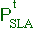,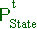= SLA and State populations respectively at time t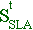,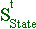= symptomatic indicator for SLA and State respectively at time t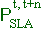= a measure of population growth in an SLA for the period t to t+n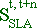= a measure of growth in the symptomatic indicator in an SLA for the period t to t+nA8.4. In the difference correlation method, growth is measured in terms of differences between proportions of the State total in the small area (for example SLA). Thus population growth between time t and t+n is expressed as=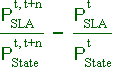(1)A8.5. Similarly, growth in the symptomatic indicator is given by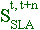=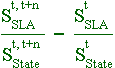(2)A8.6. The following relationship between the two growth rates:=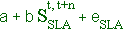(3)Where 'a' and 'b' are regression coefficients from data on all SLAs,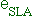is an error term, and t and t+n refer to two successive census dates.
A8.7. With this information, growth in SLA population estimates from time t+n to time T can be obtained from equations (1), (2) and (3) as follows: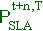=substituting for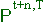from equation (1),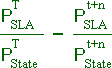=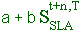or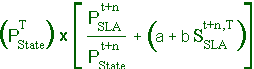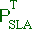= (4)where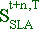is known and the coefficients a, b are estimated from equation (3).

A8.8. It should be pointed out that the difference correlation method can be easily modified to accommodate more than one symptomatic indicator. In this case, the simple linear regression equation, equation (3), is replaced by a multi-variable linear equation of the form:=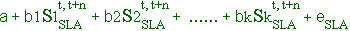where,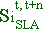refers to the ith symptomatic indicator of growth in the SLA; a, b1,......bk are regression coefficients and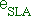is an error term.

You have reached the end of publication 3228.0

 Previous Page